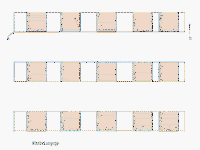# IT技术网www.itjs.cn# C++ 11 中正则表达式使用示例及源码分析

## 确保你的编译器支持Regex

```terminate called after throwing an instance of 'std::regex_error'
what():  regex_error
Aborted (core dumped)```

## regex库概览

 basic_regex 正则表达式对象,是一个通用的模板，有typedef basic_regex regex 和 typedef basic_regexwregex； regex_match 将一个字符序列和正则表达式匹配 regex_search 寻找字符序列中的子串中与正则表达式匹配的结果,在找到第一个匹配的结果后就会停止查找 regex_replace 使用格式化的替换文本，替换正则表达式匹配到字符序列的地方 regex_iterator 迭代器，用来匹配所有 的子串 match_results 容器类，保存正则表达式匹配的结果。 sub_match 容器类，保存子正则表达式匹配的字符序列.

ECMASCRIPT正则表达式语法

## 构造正则表达式

```typedef basic_regex<char>    regex;
typedef basic_regex<wchar_t> wregex;```

```//默认构造函数，将匹配任何的字符序列
basic_regex();
//用一个以‘\0’结束的字符串s构造一个正则表达式
explicit basic_regex( const CharT* s,flag_type f =std::regex_constants::ECMAScript );
//同上，但是制定了用于构造的字符串s的长度为count
basic_regex( const CharT* s, std::size_t count,flag_type f = std::regex_constants::ECMAScript );
//拷贝构造，不赘述
basic_regex( const basic_regex& other );
//移动构造函数
basic_regex( basic_regex&& other );
//以basic_string类型的str构造正则表达式
template< class ST, class SA >
explicit basic_regex( const std::basic_string<CharT,ST,SA>& str, flag_type f = std::regex_constants::ECMAScript );
//指定范围[first,last)内的字符串构造正则表达式
template< class ForwardIt >
basic_regex( ForwardIt first, ForwardIt last, flag_type f = std::regex_constants::ECMAScript );
//使用initializer_list构造
basic_regex( std::initializer_list<CharT> init, flag_type f = std::regex_constants::ECMAScript );```

 flag_type effects icase 在匹配过程中忽略大小写 nosubs 不保存匹配的子表达式 optimize 执行速度优于构造速度

```std::string pattern("http(s) ://([\\w-]+\\.)+[\\w-]+(/[\\w- ./ %&=]*) ");    //匹配规则很简单，如果有疑惑，可以对照语法查看
std::regex r(pattern);```

## 正确地处理输入

```int main()
{
std::string tmp,html;
while(std::cin >> tmp)
html += tmp;
}```

```int main()
{
std::string tmp,html;
while(getline(std::cin,tmp))
{
html += tmp;
html += '\n';
}
}```

## regex_search()只查找到第一个匹配的子序列

```template< class STraits, class SAlloc,class Alloc, class CharT, class Traits >
bool regex_search( const std::basic_string<CharT,STraits,SAlloc>& s,
std::match_results<typename std::basic_string<CharT,STraits,SAlloc>::const_iterator, Alloc>& m,
const std::basic_regex<CharT, Traits>& e,
std::regex_constants::match_flag_type flags = std::regex_constants::match_default );```

 Constant Explanation `match_not_bol` The first character in [first,last) will be treated as if it is not at the beginning of a line (i.e. ^ will not match [first,first) `match_not_eol` The last character in [first,last) will be treated as if it is not at the end of a line (i.e. \$ will not match[last,last) `match_not_bow` "\b" will not match [first,first) `match_not_eow` "\b" will not match [last,last) `match_any` If more than one match is possible, then any match is an acceptable result `match_not_null` Do not match empty sequences `match_continuous` Only match a sub-sequence that begins at first `match_prev_avail` --first is a valid iterator position. When set, causes match_not_bol and match_not_bow to be ignored `format_default` Use ECMAScript rules to construct strings in std::regex_replace (syntax documentation) `format_sed` Use POSIX sed utility rules in std::regex_replace. (syntax documentation) `format_no_copy` Do not copy un-matched strings to the output in std::regex_replace

`std::smatch results;<br>regex_search(html,results,r);`

## 使用regex_iterator匹配所有子串

```regex_iterator(BidirIt a, BidirIt b,                                                           //分别是待匹配字符序列的首迭代器和尾后迭代器
const regex_type& re,                                                           //regex对象
std::regex_constants::match_flag_type m = std::regex_constants::match_default); //标志，同上面的regex_search()中的``````#include <iostream>
#include <regex>
#include <string>

int main()
{
std::string tmp,html;
while(getline(std::cin,tmp))
{
tmp += '\n';
html += tmp;
}
std::string pattern("http(s) ://([\\w-]+\\.)+[\\w-]+(/[\\w- ./ %&=]*) ”);
pattern = “[[:alpha:]]*” + pattern + “[[:alpha:]]*”;
std::regex r(pattern);
for (std::sregex_iterator it(html.begin(), html.end(), r), end;     //end是尾后迭代器，regex_iterator是regex_iterator的string类型的版本
it != end;
++it)
{
std::cout << it->str() << std::endl;
}
}```

```[regex]g++ regex.cpp  -std=c++11 -omain
[regex]main < itjs.html

http://www.itjs.cn/program/cjc/12192LH015.html
http://www.itjs.cn/program/cjc/12111332015.html
http://www.itjs.cn/program/cjc/12111322015.html
http://www.itjs.cn/program/cjc/12111312015.html
http://www.itjs.cn/program/cjc/12111302015.html
http://www.itjs.cn/program/cjc/12111202015.html
http://www.itjs.cn/program/cjc/1211922015.html
http://www.itjs.cn/program/cjc/12111152015.html
http://www.itjs.cn/program/cjc/12111062015.html
http://www.itjs.cn/program/cjc/12111102015.html```

## regex和异常处理

 code 含义 error_collate 无效的元素校对 error_ctype 无效的字符类 error_escape 无效的转移字符或者无效的尾置转义 error_backref 无效的向后引用 error_brack 方括号不匹配 error_paren 小括号不匹配 error_brace 大括号不匹配 error_badbrace 大括号中的范围无效 error_range 无效的（不合法）字符范围 error_space 内存不足 error_badrepeat 重复字符之前没有正则表达式（* + ） error_complexity 太复杂了，标准库君hold不住了 error_stack 栈空间不足了

## 小结

C++11标准库中的正则表达式部分还有部分内容本文没有涉及，个人以为掌握了以上的内容后，基本上看一看接口就知道怎么使用了，这里就不浪费篇幅了。